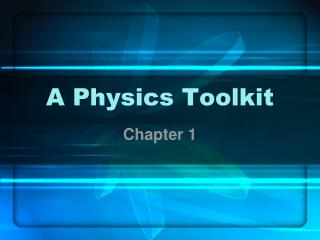Download PresentationA Physics Toolkit

A Physics Toolkit - PowerPoint PPT Presentation

A Physics Toolkit. Chapter 1. 1.1 Physics. Energy, matter and their relationship Understanding the physical world Careers Scientists, astronomers, engineers, teachers, computer science, medicine. Mathematics. Language of physics Model observations RepresentationsI am the owner, or an agent authorized to act on behalf of the owner, of the copyrighted work described.
Download PresentationA Physics Toolkit

Download Policy: Content on the Website is provided to you AS IS for your information and personal use and may not be sold / licensed / shared on other websites without getting consent from its author.While downloading, if for some reason you are not able to download a presentation, the publisher may have deleted the file from their server.

- - - - - - - - - - - - - - - - - - - - - - - - - - E N D - - - - - - - - - - - - - - - - - - - - - - - - - -
Presentation Transcript
1. A Physics Toolkit Chapter 1

2. 1.1 Physics • Energy, matter and their relationship • Understanding the physical world • Careers • Scientists, astronomers, engineers, teachers, computer science, medicine

3. Mathematics • Language of physics • Model observations • Representations • Equations, pictures, force diagrams, written descriptions, motion maps…

4. DOES IT MAKE SENSE?? • Numbers, units, blah, blah, blah • Copper ball falls 5 m. • Calculated answers: 0.002 s or 17 s

5. SI Units • 7 base units: Meter, Kilogram, Second, Kelvin, Mole, Ampere, Candela • Everything else is derived • Joule • Coulomb • Newton

6. Conversions • Femto (10-15) to tera (1012) • Same as chemistry • Dimensional analysis • 1kg/1000g = 1

7. Significant Figures • Zeros to locate the decimal are not significant • Scientific notation makes it easier to identify significant zeros • Arithmetic result may never be more precise than the least precise measurement • Addition, subtraction, multiplication, division • Counting numbers are exact

8. Scientific Methods • Make observations • Do experiments • Create models or theories to explain results • Repeat for many iterations • How are variables related?

9. MODELS, Laws, Theories • Models based on experimentation • Atomic model, Bohr model, Quantum model • New data that doesn’t fit existing model • Evaluate both data and model • Reproducibility of data • Validity of model

10. Models, LAWS, Theories • Rule of nature • Sums up related observations • Describes pattern in nature • Conservation laws, Law of reflection • Do NOT explain why things happen

11. Models, Laws, THEORIES • Explanation based on many observations • Based on experimental results • May be explanations of laws • Only a well supported explanation • Theory of Universal Gravitation, Atomic Theory

12. 1.2 Measurement • Comparison between a standard and unknown quantity • Often reported with uncertainty • If values overlap within uncertainty, data agrees • Figure 1-10, pg 12

13. PRECISION versus Accuracy • Degree of exactness of measurement • Smallest amount of uncertainty is most precise • Depends on instrument and technique • Finest division on device • Precision is ½ smallest division • Graduated cylinder with 1 mL division • Beaker with 50 mL division • Shown by significant figures

14. Precision versus ACCURACY • Describes how well measurements agree with the accepted value • Real value versus Accepted value • Can you ever know the exact measurement? • Yes and no

15. Good Measurements • Read measurements at eye level • Reduces error caused by parallax • Carefully • Multiple times as necessary • Correct device for type of measurement

16. 1.3 Graphing Data • Represent relationships between variables • Independent variable is manipulated • Dependent variable responds • Rule of One

17. Line of Best Fit • Best model of the data • Drawn close to all data points possible • Problem-solving strategies, pg 16

18. Linear Relationships • Dependent variable varies linearly with independent variable • Relationship is y=mx+b • Use points on the line of best fit to calculate slope and y-intercept • Slope = change in y over change in x

19. Nonlinear Relationships • Quadratic • y = ax2 + bx + c • One variable depends on the square of another • Inverse (hyperbola) • y = a/x • One variable depends on the inverse of the other • Others

20. Predicting Values • Can use the graph to make predictions • Interpolation • Extrapolation • Can use the equation for the graph to make predictions

21. Lab • Graphing activity – after finishing slides • Vernier Caliper lab – after section 2 discussion